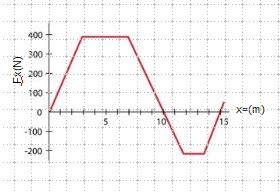# Determine the work done by this force to move the particle along the x axis from x=0.0 to x=10.0m;

The force on a particle, acting along the x axis, varies as shown in the figure below.

Part A Determine the work done by this force to move the particle along the x axis from x=0.0 to x=10.0m;
Part B Determine the work done by this force to move the particle along the x axis from x = 0.0 to x = 15.0m.